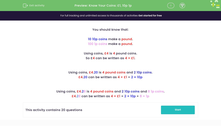# Partition money into £1, 10p and 1p coins

In this worksheet, students express sums of money in multiples of £1, 10p and 1p coins.Key stage:  KS 2

Curriculum topic:   Measurement

Difficulty level:#### Worksheet Overview

In this activity, we will be learning how to make amounts in pounds and pence using different coins.

It is useful to remember the following facts:

Ten 10p coins = £1

One hundred 1p coins = £1

Using coins, we can make £4 using four £1 coins.

So £4 could be written as 4 × £1.

Using coins, we can make £4.20 with four £1 coins and two 10p coins.

So, £4.20 can be written as 4 × £1 + 2 × 10p

To make £4.28, we would need to use four £1 coins, two 10p coins, and eight 1p coins.

So, £4.28 can be written as 4 × £1 + 2 × 10p + 8 × 1p

Let's try an example question.

Example

Which coins could be used to make £2.24?

To make £2.24, we would need two £1 coins.

We would also need to make 24 pence, which could be done in several ways.

Here is one way: one 20p coin and four 1p coins

So, £2.24 could be written as 2 × £1 + 1 × 20p + 4 × 1p